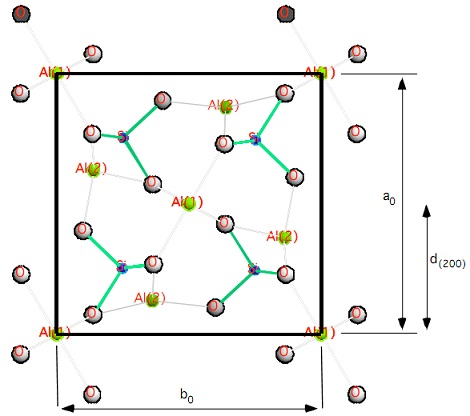Bragg equation-room temperature d-spacing

An X-ray diffraction laboratory is analyzing the mineral andalusite (Al2SiO5). Powder diffraction of andalusite is being done while using a heating stage to heat the sample. Powder patterns are collected for andalusite at room temperature (25oC), as well as at 400, 600, and 800 oC.

1) It is observed that the XRD powder pattern for andalusite changes as temperature increases. Using the Bragg equation (assuming n = 1) and that Cu Kα radiation was used (where λ = 1.541 Å): What is the room temperature d-spacing for the (200) peak at 2θ = 22.810o ?

Please calculate to 3 decimal places.

2) Using the same experimental conditions as above, calculate the d-spacing for the (200) peak at 400 oC, where 2θ = 22.710o; at 600 oC, where 2θ = 22.640o; and at 800 oC, where 2θ = 22.580o. Please calculate to 3 decimal places.

3) What is happening to the a0 dimension of the unit cell of the andalusite crystal structure, when it is being heated? Plot the a0 (a-axis) unit cell dimension  (to 3 decimal places)  versus temperature (oC).
a0 = 2 * d(200)Figure: C-axis projection of the unit cell of the andalusite structure. The unit cell boundary is drawn as thick lines.